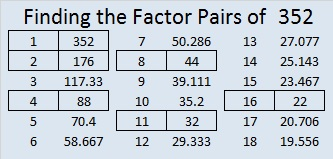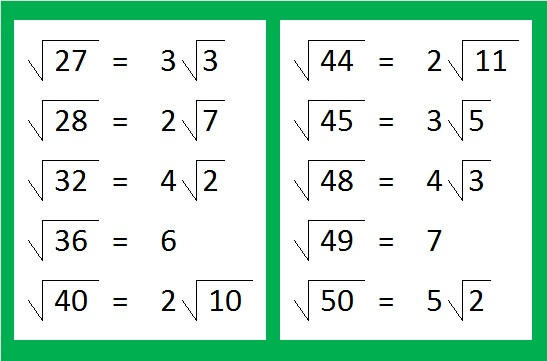# Square Roots up to √352 That Can Be Simplified

• 352 is even and therefore a composite number.
• Prime factorization: 352 = 2 x 2 x 2 x 2 x 2 x 11, which can be written 352 = (2^5) x 11
• The exponents in the prime factorization are 5 and 1. Adding one to each and multiplying we get (5 + 1)(1 + 1) = 6 x 2 = 12. Therefore 352 has exactly 12 factors.
• Factors of 352: 1, 2, 4, 8, 11, 16, 22, 32, 44, 88, 176, 352
• Factor pairs: 352 = 1 x 352, 2 x 176, 4 x 88, 8 x 44, 11 x 32, or 16 x 22
• Taking the factor pair with the largest square number factor, we get √352 = (√16)(√22) = 4√22 ≈ 18.762Mathematics is full of interesting patterns. Let’s explore some patterns in reducible square roots.

Here are the first ten numbers that have reducible square roots. Notice that five of the numbers in this list, 1, 4, 9, 16, and 25 are perfect squares:40% of the numbers up to 5 have reducible square roots. The same thing is true for 40% of the numbers up to 10, 40% of the numbers up to 20, and 40% of the numbers up to 25.

Here are the second ten numbers with reducible square roots. The last number,50, is double the previous last number, 25. Again 40% of the numbers up to 50 have reducible square roots, and only two of these numbers are perfect squares. Notice that the last three numbers under the radical sign in this set are consecutive numbers.Would you like to make any predictions for the third set of ten numbers with reducible square roots?

If you predicted that this set of numbers would end with 75, you were almost right! 29/75 or 38.67% of the numbers up to 75 have reducible square roots, and 30/76 or 39.47% of the numbers up to 76 do. Both of these values are very close to 40%, but not quite there. Notice this time only one number is a perfect square.Would you like to make a prediction for what will happen with the fourth set of ten numbers with reducible square roots?

Surprise! We’re back to 40% of the numbers up to 100 have reducible square roots. Those consecutive numbers at the end of the set really helped raise the percentage right at the last minute. This set of numbers has two perfect squares.What do you think will happen if we look at all the reducible square roots up to 350? Multiples of any perfect square will always have reducible square roots. Since we have more perfect squares, do you think more will be reducible?

Because the square roots of 351 and 352 are also reducible, let’s include them in this chart. Each column has 20 reducible square roots in it, and they are grouped into fives for easier counting. I’ve highlighted sets of three or four consecutive numbers. In all, we now have charts showing the first 140 reducible square roots. This last set has eight perfect squares. Let’s look at the percentages at the end of some of those sets of consecutive numbers: 50/126 or 39.68% of the first 126 numbers have reducible square roots. 96/245 or 39.18% of the first 245 numbers have reducible square roots. Finally, 140/352 or 39.77% of the numbers up to 352 have reducible square roots. That one is so close to 40%!

No matter how big a table we make, the percentage of reducible square roots will be very close to 40%. It will not get significantly higher because, believe it or not, most numbers are either prime numbers or the product of two or more DIFFERENT primes and thus have square roots that are NOT reducible.

1.wbhs62
•ivasallay